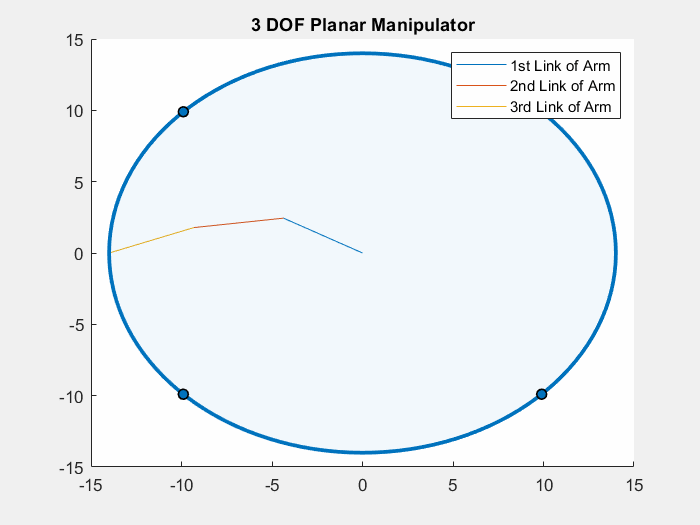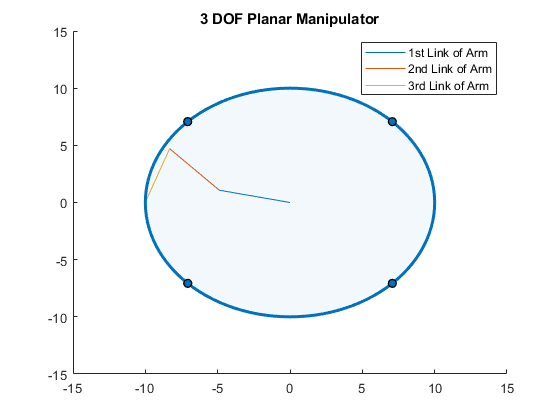Author: Yash Bansod
GitHub: https://github.com/YashBansod

This is the main program.

## Clear the environment and the command line

``````clear;
clc;
close all;
``````

## Add the directory containing relevant functions to the path variables

``````addpath('./INV-functions/')
``````

## Define the input parameters and simulate

``````% Set the length of the links of the manipulator robot.
L1 = 5;
L2 = 5;
L3 = 5;

% Set the initial orientation of the robot.
theta1 = 10;
theta2 = 0;
theta3 = 15;

% Define the radius of the circle the end effector should follow

hold on;
% Code for drawing a circle
expX = i;
expY = sqrt(r_sq - expX^2);
cla;
% You can modify the Kp value defined in PLANAR_INV_KIN_3DOF to modify
% the inverse jacobian controller behavior.
[expPoint, Joint, Theta] = PLANAR_INV_KIN_3DOF(L1, L2, L3, expX, ...
expY, theta1, theta2, theta3);
scatter(Joint(end,1), Joint(end,2));
theta1 = Theta(1, 1);
theta2 = Theta(2, 1);
theta3 = Theta(3, 1);
end

expX = i;
expY = -sqrt(r_sq - expX^2);
cla;
% You can modify the Kp value defined in PLANAR_INV_KIN_3DOF to modify
% the inverse jacobian controller behavior.
[expPoint, Joint, Theta] = PLANAR_INV_KIN_3DOF(L1, L2, L3, expX, ...
expY, theta1, theta2, theta3);
theta1 = Theta(1, 1);
theta2 = Theta(2, 1);
theta3 = Theta(3, 1);
end
``````

## Results